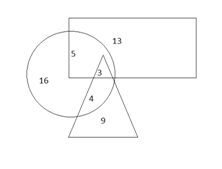# SSC CGL 2018 Practice Test Papers | Quantitative Aptitude (Day-19)

Dear Aspirants, Here we have given the Important SSC CGL Exam 2018 Practice Test Papers. Candidates those who are preparing for SSC CGL 2018 can practice these questions to get more confidence to Crack SSC CGL 2018 Examination.

[WpProQuiz 2937]

Click “Start Quiz” to attend these Questions and view Explanation

1) Two numbers A and B are in the ratio 7:5. Their LCM is 105. Find the HCF.

a) 1

b) 8

c) 3

d) 2

2) A train passes a pole in 8 seconds and a railway bridge of 180 meter length in 20 seconds. Find the length of the train?

a) 120

b) 240

c) 200

d) 180

3) The cost price of a fridge is 10200. It is sold to a customer at two successive discounts of 20%. Find the selling price of the fridge?

a) 7500

b) 6528

c) 6120

d) 8000

4) A man can row 18 km/h upstream and 24 km/h downstream. Find the speed of boat?

a) 21 km/h

b) 3 km/h

c) 20 km/h

d) 10 km/h

5) There are 2000 students in a school. 60 % of the students come to school by bus.  3/5 of those come by bus are boys. Find the no. of girls come to school by bus.

a) 240

b) 300

c) 600

d) 480

6) A and B working together can do a piece of work in 6 days. A alone can do the work in 18 days. Find the number of days taken by B to complete the remaining work if 1/3 of work is already done by A

a) 4

b) 10

c) 12

d) 6

7) The value of m(p+q)÷mp÷m-pis________

a)mq

b)m(p+q)

c)mp

d)m( p-q)

Direction : (8- 10) Refer the below diagram and answer the following questions.

In a class of 50 students, the number of students passed in Maths is represented as Circle and the number of students passed in English is represented as Rectangle and the number of students passed in Economics is represented as triangle.8) What is the number of students passed in more than one subject?

a) 5

b) 3

c) 8

d) 12

9) What is the difference between the number of students passed in Maths alone and the number of students passed in all three subjects?

a ) 13

b) 8

c) 9

d) 4

10) What is the sum of number of students passed in Economics and English?

a) 37

b) 51

c) 34

d) 41

Let A and B be 7x and 5x respectively.

If A= 7x, B= 5x, then HCF =x

7x × 5x=35 x2

Product of 2 numbers= LCM x HCF

35 x2 =105 * x

x= 3

Length of train=x

x/8 = (x+180)/20

5x=2(x+180)

3x=360

X=120 m

80/100×80/100x 10200= 6528

Upstream speed, x= 18 km/h

Downstream speed, y= 24 km/h

Speed of boat = (x+y)/2 = (18+2)/2 =21 km/h

Total students= 2000

Students come by bus= 60/100 x2000= 1200

No. of girls come by bus= 2/5 x1200= 480

A+B= 1/6

A=1/18

B=1/ 6-1/18=1/9

Time taken by B to complete 2/3 of work = 9 x (2/3)=6 days

m(p+q)÷mp÷m-p=m( p+q)

5+3+4=12